Rectangular Coordinates

Sol A The circle has an equation of the formor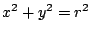where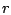is the distance from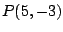to the origin; soand the circle has the equation.

Sol B The midpoint of the line segment AB is given by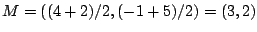, so the circle has the equation.

Sol 1 The midpoint of the line segment AB is given by, so the distance from P to M is equal to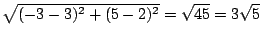.

Sol 2 The radius of the circle is the distance from C to the x-axis, so. Therefore the circle has equation.

Sol 3 The circle has an equation of the form, where the radiusis the distance from C to P. Instead of using the distance formula, though, we can substitute the coordinates of P into the equation to getor.

Sol 4 The radius of the circle is the distance from C to the line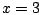, so. Therefore the circle has equation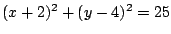.

Sol 5 The center C of the circle is the midpoint of the line segment between P and Q, so. Therefore the circle has an equation of the form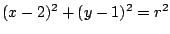, whereis the distance from C to P (or Q). Instead of findingfirst, though, we can substitute the coordinates of P into this equation to getor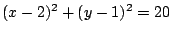.

Sol 6 First we can find the center and radius of the circleby completing the square:gives, so the circle has center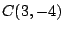and radius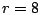. The distance from P to C is given by. Since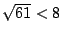, the point P is inside the circle.

Sol 7 The center of the circle is the point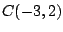, and the slope of the line through P and C is given by. Therefore the line through P and C has the equation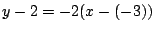or, and the point on the circle closest to P will be one of the points of intersection of this line with the circle. Substitutinginto the equation of the circle gives, so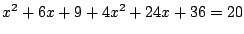or. Then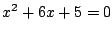, soandor. Since the x-coordinate of P is 1, the point on the circle closest to P hasand; so it is the point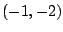.Sol 8 First we will find an equation of the line through P which is perpendicular to the given line. Solvingforgives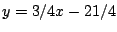, so the given line has slope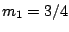and therefore a line perpendicular to this line will have slope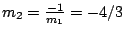.

Thus the line through P perpendicular to the given line has equationor. These two lines will intersect at a point Q which is the point on the given line closest to P, and we can find the coordinates of Q by substitutinginto the equationand then solving: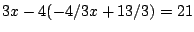gives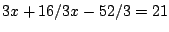or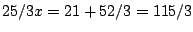, soand. Therefore Q is the point,

and the distance from P to the line is the distance from P to Q, which is given by.